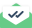# Thermodynamic properties

Dear Lammps users,

excuse me because of directly emailing you, I am confused and there is nobody in lammps forum to help me. I used Lammps for investigating the accuracy of an equation of state for different ionic liquids. My simulations predict the PVT data of the substances very accurate compared to experimental data.

actually, the experimental data for thermodynamic properties of ionic liquids that I used are very scarce, therefore, I should compare the total energy and enthalpy with the PVT data which are proved to be correct in compared to experimental data and I used a formula from my equation of state. total energy and enthalpy which are in Lammps log file are very different from E, H that I calculate from Lammps log file PVT. I am sure about the formula of the equation of state and unit conversion.

these are from my input file:

units real
dimension 3
boundary p p p
atom_style full

thermo_style custom step temp etotal ke pe press vol enthalpy

I used NPT and NVE ensembles, but E,H are from NPT.

what are the enthalpy and total energy in lammps log file?
how can I compare this with enthalpy function [{ (H(T) - H(0)) /T*} J/K/mol]? ( H(0) = enthalpy in 0 K and 0 kPa )Sent with Mailtrack

Anytime you are comparing thermodynamic energies, you have to be careful, because there are many choices of reference state. In addition, the thermo quantities calculated by LAMMPS, such as temperature and enthalpy, are instantaneous values. They are only equivalent to the corresponding thermodynamic properties when sampled over a suitably long simulation and averaged. The LAMMPS doc page for the thermo_style command explains that enthalpy is defined as

enthalpy = enthalpy (etotal + press*vol)

etotal is the sum of kinetic energy (from the particle velocities) and potential energy (from pair_style, kspace, and whatever else you have going on)

The units of enthalpy are kcal/mol if real units are used. But mol here means moles of your system. So if your system contains 64 molecules, you will have to divide by 64 to get something that you can compare to an experimental value.

Now, what about the reference state? Nobody does experiments at T=0, or even attempts to extrapolate to that state. Instead, they pick some convenient ambient state, which could be that of the pure elements making up the molecules (oxygen gas at STP, et cetera). Unless you are trying to explicitly simulate reactions, you don’t care about that. So maybe you are interested in the enthalpy change on heating from 25 C to 100 C. If that is the case, just run LAMMPS with NPT at 298K and 373K, P=1atm in both cases, the difference in the average enthalpies is your answer.

Aidan